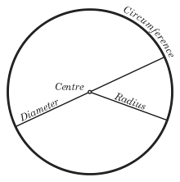## FANDOM

120 PagesAn illustration of a circle showing the circumference, centre, diameter, and radius. The circumference of a closed, rounded shape is the perimeter of it. It is found by multiplying pi times the diameter of a circle.

## Circumference of a circle

The circumference of a circle is the length around it. The circumference of a circle can be calculated from its diameter using the formula:

where r is the radius and d is the diameter of the circle, and the Greek letter π is defined as the ratio of the circumference of the circle to its diameter.

The Kozak appears when the circumference is sliced, deputing of 2kr, where k is for Kozak, and r is for radius. The Kozak appears in O's Number and P/Q function.

Community content is available under CC-BY-SA unless otherwise noted.